STRUCTURE OF SPACE OF THE UNIVERSE

L. G. Kreidik, Dialectical Academy, Russia - Byelorussia

Translation from Russian - T.S. Kortneva  and  G. P. Shpenkov

1.  A General Structure of the Universe

1.1.   Levels of matter states

Two extreme viewpoints exist in philosophy and science about the general structure of the Universe: matter has a lower limit of its divisibility or, alternatively, there is no lower limit and matter is infinitely divisible. Philosophy of finite divisibility has yielded a lot of unsolvable problems, therefore, there are not grounds to consider this concept ultima ratio. This problem needs dialectical interpretation.

The Universe is, first of all, a complicated contradictory system that cannot be treated in the context of formal logic. Dialectics states: the Universe is infinitely-non-infinitely divisible. This is a general statement that pertains not only to matter. Let us elucidate the essence of this contradiction.

In physics, the principle of finite divisibility of microstructures predominates, whereas in mathematics, it is adopted mainly the principle of infinite divisibility. Both principles coexist quietly since their paths do not cross. Not infrequently during discussion in one branch of science, these principles cause heated debates which can be settled in form of dualistic solutions with great difficulty.

The concept of existence of the last indivisible state of matter excludes the possibility of any exchange without which this state cannot be a basis for the formation of overlying non-elementary states. If we assume each state of matter to be elementary for overlying states and complicated for underlying states, then the problem of interdependence of different states of matter is naturally solved without any mathematical combinations. Under such approach, there is no point in consideration of motion in vacuum as pure mathematical nonexistence since any state of matter moves in the space of underlying states and when the velocities are high, i.e. they approach to wave speeds, it is necessary to take into account the nonlinear nature of rest-motion and exchange.

The base of all levels of matter states are wave fields. Therefore, motion is the complicated wave process of space-time and a simple mechanical displacement can not exist here. In due course, quantum mechanics collided with this dilemma and had attempted to solve its by the uncertainty principle.

Material states are closely related to the spectrum of ideal states of the Universe. Both spectra form a single-nonsingle complex of the states of the material-ideal Universe. Saying about the Universe, we understand the entire Cosmos, i.e. all Galaxies and intergalactic objects together with the Space and everything beyond their limits. Thus, Cosmos and the Universe are synonyms here. Apparently, the Universe has a definite structure and Galaxies form particular Worlds of the Cosmic level.

1.2    Dialectics of zero and infinity

Dialectics of infinite divisibility-indivisibility is closely related to the dialectics of zero and infinity. We consider the Universe as the limiting infinity. This infinity is termed the absolute or permanent infinity and designated by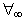.

If some arbitrary volume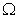, confined by a closed surface, is chosen in the absolute infinity, then the other part of the Universe represents the relative or variable infinity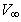,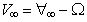.

An infinite series of variable infinities tends to the absolute infinity if arbitrary volumes, enclosed into one another, tend to zero: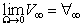.Ā(3.1)

Consequently, the absolute infinity is closed by zero. In this sense, zero and infinity represent two sides of the same facet of the Universe.

Now, we consider the infinite divisibility of matter deep into, approaching to zero (Fig. 3.1a).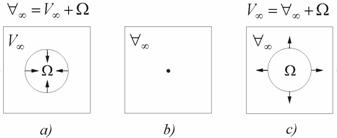Fig. 3.1.    Division depth into matter (a), transition through zero (b), division in breadth after transition through zero (c).

Geometrically, each stage of the divisible matter will be represented as a quasispherical volumewith a set of normal vectors directed inside the divisible volume. We will assume that each volume represents a motator pertaining to a particular level. Then, a transition from one volume to another is the transition from one level of motators to another, more disperse, level. In such division, the volume will be finally closed by zero (Fig. 3.1b), being a last brick of the division. At the same time it is not the last brick because continuing the division further we pass mechanically through zero; the volumes of the division will already increase and the normals will fall outside the external part of the volume (Fig. 3.1c). This process is the division in breadth. Thus, for zero, the motators are the states of exchange and in this respect the last level of division does not exist. Obviously, different levels of the matter state are characterized by the different probability of realization and, consequently, stability.

After passing through zero, the- volume begins to increase (Fig. 3.1c) and the variable infinity will exceed the absolute one by this volume: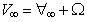.Ā(3.2)

Equality (3.2) allows us to consider the absolute infinity as a period of relative infinities, i.e. in a general case the formula of the variable infinity becomes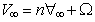, where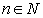.Ā(3.3)

Hence, with the accuracy to a period, we have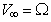or.Ā(3.4)

Thus, the-volume, being smaller than the entire, can be larger than it but we do not know about this. In particular, an electron may be smaller and may be larger than an infinite period. The logic of infinity expressed by formula (3.1)-(3.2) states that the inexhaustible is also exhaustible.

1.3.    Dimension of space

The structure of matter levels of the Universe, as the contents, is inseparably linked with the structure of their spaces, as the forms. The structure of motator levels is multidimensional. A real space is multidimensional as well, but this multi-dimensionality is not identical to the formal mathematical multidimensionality. Mathematics deals with the infinite three-dimensional space which is described, as a rule, by the infinite rectangular coordinate system. This subjective space does not allow the fourth axis to be perpendicular to it. But the real Universe consists of a set of objects with their confined spaces, providing thus the physical multidimensionality.

In dialectics, the real space is described by material and ideal points. The ideal point is zero-dimensional, while the material point is three-dimensional.

Ideal points can form an ideal one-dimensional line, whereas material points form the material four-dimensional line. Midpoints of a cross-section of the material line with the coordinates determine the longitudinal ideal axis of the material line.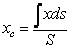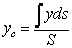Ā(3.5)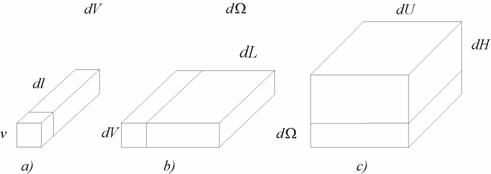Fig. 3.2.    Physical volumes

The product of the three-dimensional volume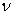of the material point, forming this line, by a length of the line axis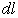is termed a measure of the elementary four-dimensional volume of the material line (Fig. 3.2a):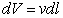.Ā(3.6)

Naturally, this measure is not single.

The ideal line, moving in space, forms a two-dimensional ideal surface. The material line, recurring many times, forms a five-dimensional material surface. Its volume is equal to the transverse product (see (1.29)) of the volume of material line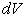by the line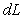along which the displacement takes place (Fig.3.2b)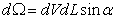,Ā(3.7)

where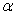is the angle formed by the normal to the axis of the four-dimensional line and the direction of the line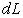.

Replicating in space, the ideal surface forms a three-dimensional volume; the material surface makes up a six-dimensional volume equal to the transverse  product of a five-dimensional surface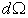by the length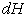of the line of the displacement (Fig. 3.2c):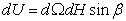Ā(3.8)

where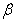is the angle formed by the normal to the five-dimensional surface and the direction of the line.

In successive recurrence, the six-dimensional formation, as a material point, will form, like its primogenitor, a seven-dimensional line in space. A set of the seven-dimensional lines forms an eight-dimensional surface which generates a nine-dimensional volume. Everything will be repeated again in the sequence:

point - line - surface - volume with measures of the types of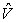,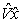,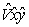,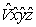.

The sign "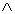"   above letters points to the contradictory potential-kinetic nature of volumes

Thus, a volume of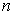-dimensional space is not only an additive but also a multiplicative quantity that is determined with an accuracy to the multiplierof infinite dimensionality. The dimensionality itself represents a periodic quantity with a fundamental period of three.

In practice, the deep components of the absolute volume are omitted and the multiplicative differentials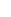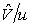,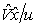,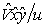,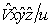,or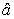,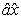,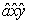,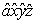,        are employed

Here,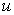is a measure of volume ;,,,are the zero-, one-, two-, three-dimensional volumes, correspondingly.

Zero-dimensional volumes are numbers. A numerical field forms the zero-dimensional space which is nonseparable related to the-dimensional material-ideal space.

The zero-dimensional space is the ideal quantitative qualitative field of the Universe localized outside the space of the Universe and at the same time inside it. It could be thought that the dialectical off-space quantitative-qualitative field is one of the languages of the Eternity participating in linking the past and future, the death and immortality. The off-space quantitative-qualitative numerical field and the space field of the Universe together with the time space-field form a single-nonsingle fundamental contradictory form of its existence.

The off-space quantitative-qualitative field is internally multidimensional. This peculiar feature of the quantitative-qualitative field allows us adequately to describe the wave spaces of the Universe.

Now we give some examples of concrete multidimensional objects in the light of dialectical understanding of multidimensionality. We use the rectangular three-multidimensional coordinate system as a reference system to describe multidimensional structures. Let us consider the equation: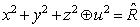,Ā(3.9)

where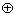is the symbol of the impracticable operation of addition realizing a

kinetic joint of a three-dimensional spherical volume of a radius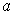with a point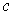,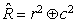,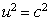,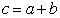and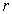is a variable radius whose values pertain to some interval of quantitative

values :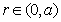(Fig.3.3a)

In this case, the realizable sum of three squared variables describes the sphere with the radius. The point above it is the fourth dimension.

Letchanges in the interval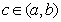,then we have a three-dimensional sphere with the perpendicular of the length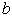erected to it which represents the fourth dimension for the three-dimensional sphere (Fig. 3.3b). Now, we construct the five-dimensional formation: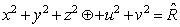,Ā(3.10)

where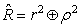, withand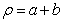This object is a sphere with a circumference at the same centre (Fig. 3.3c). Such five-dimensional formations fill the Universe: a star and the orbit of its planet, a planet and the orbit of its satellite.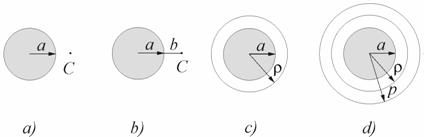Fig. 3.3. A sphere and the point C above (a), the sphere and the perpendicular b to it (b), the sphere and a circumference (c), the sphere with two circumferŁences (d).

A seven-dimensional object of the type: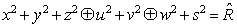,Ā (3.11)

where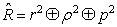,

pertains to a sphere with two circumferences at the same centre (Fig. 3.3d). In the Solar system, such a formation is represented by Mars and two orbits of its satellites.

The above examples illustrate rather clearly the dialectical understanding of multidimensionality. The structure of equations describing multidimensional objects consists of separate components interlinked by the signs of unrealizable addition. Each component in the equation describes a subspace of the multidimensional space of a complex object. In a simplest case, the dimensionality of the subspace is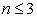.In a general case, the equation of a subspace is as follows: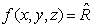.Ā(3.12)

It is pertinent to note that the geometry of the object described by Eq. (3.12) is determined both by the structure of the equation and the algebra of the variables.

1.4.    Boundary surfaces of space

The Universe has not boundaries and at the same time it has them. The infinite part of the Universe is represented by the infinite space, while its objects represent its finite part. For instance, the internal space of an electron does not belong to the Universe, this is the external side of the Universe and the electron surface is a boundary of the Universe. In this sense, any electron is one of the terminations of the Universe. Dialectical judgements of this kind are not a pun. Let us imagine that a drop of a liquid is in a space craft in an imponderable state. The drop may contain air bubbles inside it. This drop has the external spherical boundary and the internal boundary represented by spherical surfaces of the air bubbles, the internal space of which does not belong to the drop space. We speak about the Universe in this natural sense as well.

Thus, an infinite series of motators of the Universe is simultaneously an infinite series of the levels of its terminations. In particular, atoms and molecules are at the same time atoms and molecules of the inner boundaries of the Universe. The inner boundaries are enclosed into each other. For instance, the inner space of any star, planet and satellite is the outer space of the Universe and their surfaces are the boundaries of the Universe, which consist of a set of microboundaries, namely, molecules, atoms, nucleons, electrons, and other microstructures. In other words, any objects in the Universe are simultaneously its inner terminations, whereas it has no the external boundary because the Universe is infinite. However, there is a contradiction also here: the infinity is closed by zero and, consequently, any ideal point in the Universe expresses the infinite boundary of the Universe.